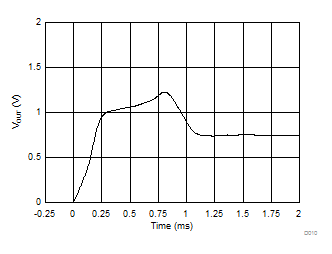ZHCSH80E September   2017  – May 2019

PRODUCTION DATA.

1. 特性
2. 应用
3. 说明
1.     Device Images
4. 修订历史记录
5. Pin Configuration and Functions
6. Specifications
7. Detailed Description
8. Application and Implementation
1. 8.1 Application Information
2. 8.2 Typical Application
1. 8.2.1 Connection to an ADC
9. Power Supply Recommendations
10. 10Layout
11. 11器件和文档支持
12. 12机械、封装和可订购信息

• DCK|5
• DBZ|3
• DCK|5

### 6.6 Typical Characteristics

at TA = 25°C, (unless otherwise noted)TMP235: VDD = 2.3 to 5.5 V, IOUT = 0 µA, CLOAD = 1000 pF
Figure 1. Accuracy vs. TA Temperature (A2 Accuracy Level)IOUT = 0 µA, CLOAD = 1000 pF
Figure 3. Output Voltage vs. Ambient TemperatureTMP235: IOUT = 0 µA, CLOAD = 1000 pF
Figure 5. Supply Current vs. TemperatureTMP235: VDD = 2.3 to 5.5 V, IOUT = 0 µA, CLOAD = 1000 pF
Figure 7. Line Regulation (Δ°C / ΔVDD) vs. Ambient TemperatureTMP235: TA = 25°C
Figure 9. Output vs. Settling Time to Step VDDTMP235: 1 × 1 (inches) PCB, Air 26°C to Fluid Bath 123°C
Figure 11. Thermal Response (Air-to-Fluid Bath)TMP235: VDD = 2.3 to 5.5 V, IOUT = 0 µA, CLOAD = 1000 pF
Figure 2. Accuracy vs. TA Temperature (A4 Accuracy Level)TMP235: IOUT = from 0 µA to 100 µA, CLOAD = 1000 pF
Figure 4. Changes in Accuracy vs. Ambient Temperature (Due to Load)TMP235: VDD = 2.3 V, CLOAD = 1000 pF
Figure 6. Load Regulation vs. Ambient TemperatureTMP235: TA = 25°C
Figure 8. Output Voltage vs. Power SupplyTMP235: TA = 25°C, VDD Ramp Rate = 5 V/ms
Figure 10. Output vs. Settling Time to Ramp VDDTMP235: TA = 25°C, VDD = 5 V, IOUT = 100 µA
Figure 12. Output Impedance vs. Frequency